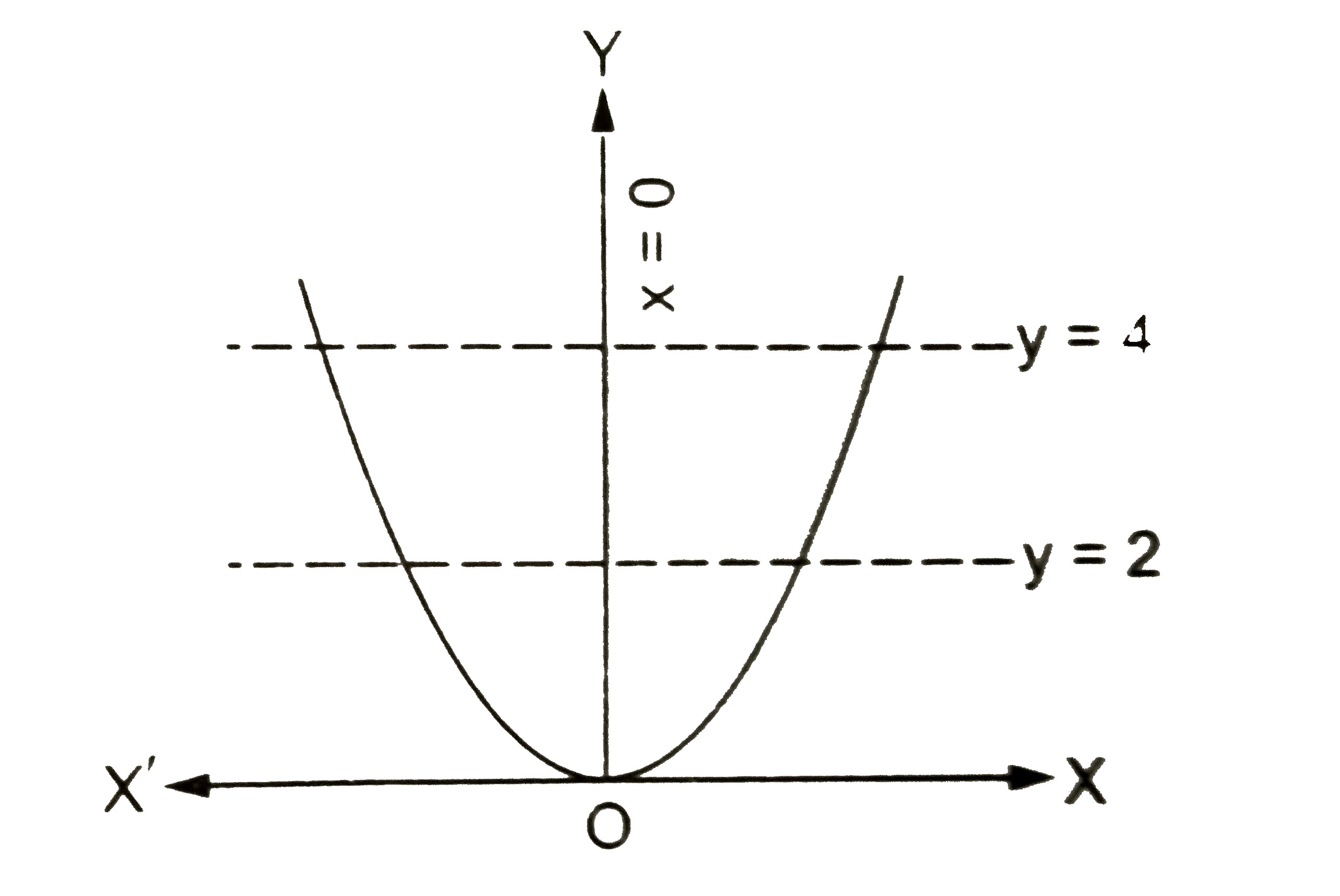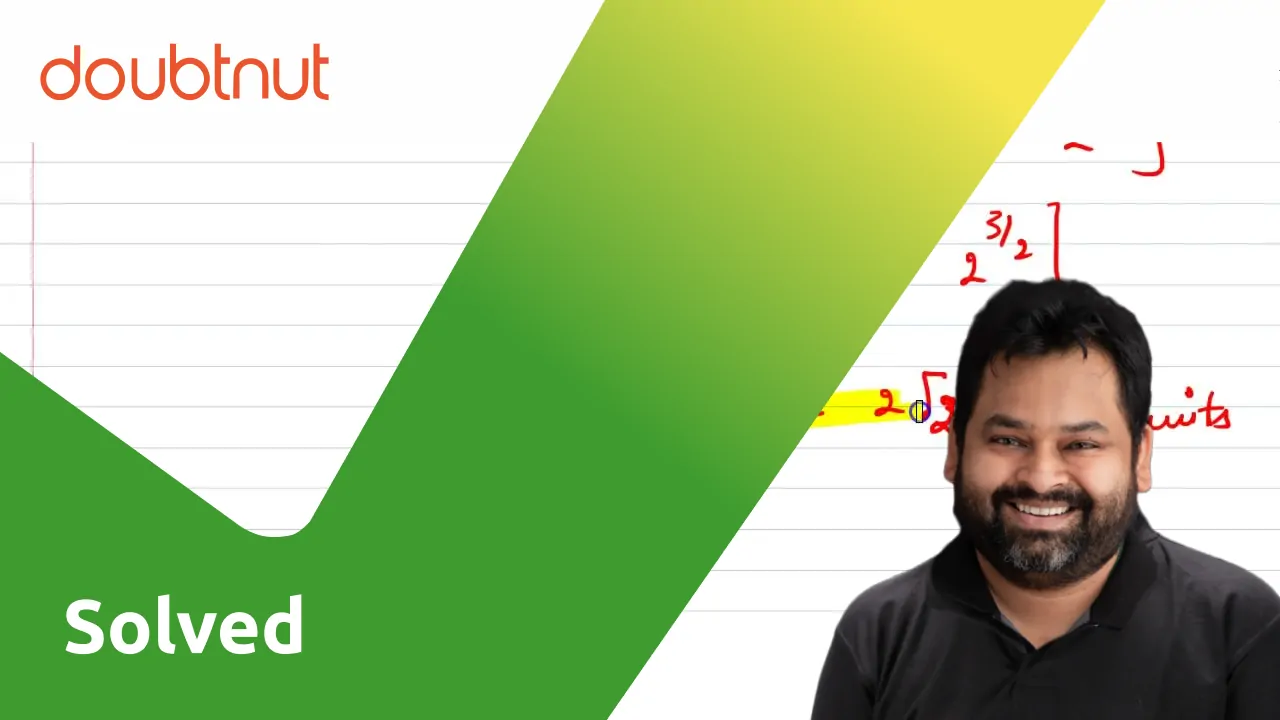Doubtnut App - Get 50 Lakh+ video solutions better than GoogleInstall Now

# Sketch the region lying in the first quadrant and bounded by y=4x2 ,x = 0, y = 2 and y = 4. Find the area of the region using integration.

Text Solution
Verified by Experts

## Clerly ,we have to find the ara the region bounded by the curve y=42 , the y-axis ans the lines y=2 and y=4 Required area 4∫2x dy=4∫212√yd cy